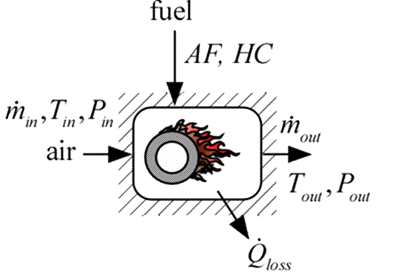Contents - index

Combustor1_CLProcedure Combustor1_CL is a model of a combustor such as used in gas turbine systems.  This model does not do combustion calculations, but rather, it accepts the heat of combustion of the fuel and the air-fuel ratio.  Combustor2 is a similar model but it does the combustion calculations.

Inputs:

T_in:  inlet temperature (K, C, F, or R)

P_in:  inlet pressure (bar, atm, Pa, kPa, MPa)

m_dot_in:  inlet mass flow rate (kg/s or lb_m/hr)

F\$:  fluid string identifier  (either Air or Air_ha)

AF:  air-to-fuel ratio

HC:  heat of combustion of fuel (J/kg, kJ/kg or Btu/lb_m)

eta:  First Law efficiency based on the provided value of HC

DPoverP:  pressure loss in combustor normalized by inlet pressure

Outputs

m_dot_out:  outlet mass flow rate (kg/s or lb_m/hr)

P_out:  outlet pressure (bar, atm, Pa, kPa, MPa)

T_out:  outlet temperature (K, C, F, or R)

Q_dot_f:  heat transfer to fuel (W, kW or Btu/hr)

Q_dot_loss:  heat transfer lost to ambient (W, kW, or Btu/hr)

Example:

\$UnitSystem SI Mass J K Pa

T_in=400 [K] "inlet temperature"

P_in=200000 [Pa] "inlet pressure"

m_dot_in=0.05 [kg/s] "mass flow rate"

F\$='Air' "fluid"

AF=30 [-] "air-fuel ratio"

HC=lowerheatingvalue(C3H8) "heat of combustion"

eta=0.97 "efficiency"

DPoverP=0.02 "nondimensional pressure drop"

Call combustor1_cl(T_in, P_in, m_dot_in, F\$, AF, HC, eta, DPoverP: m_dot_out, T_out, P_out, Q_dot_f, Q_dot_loss)

{Solution:

{m_dot_out = 0.0516 [kg/s]

T_out = 1666 [K]

P_out = 196000 [Pa]

Q_dot_f = 74900 [W]

Q_dot_loss = 2316 [W]}